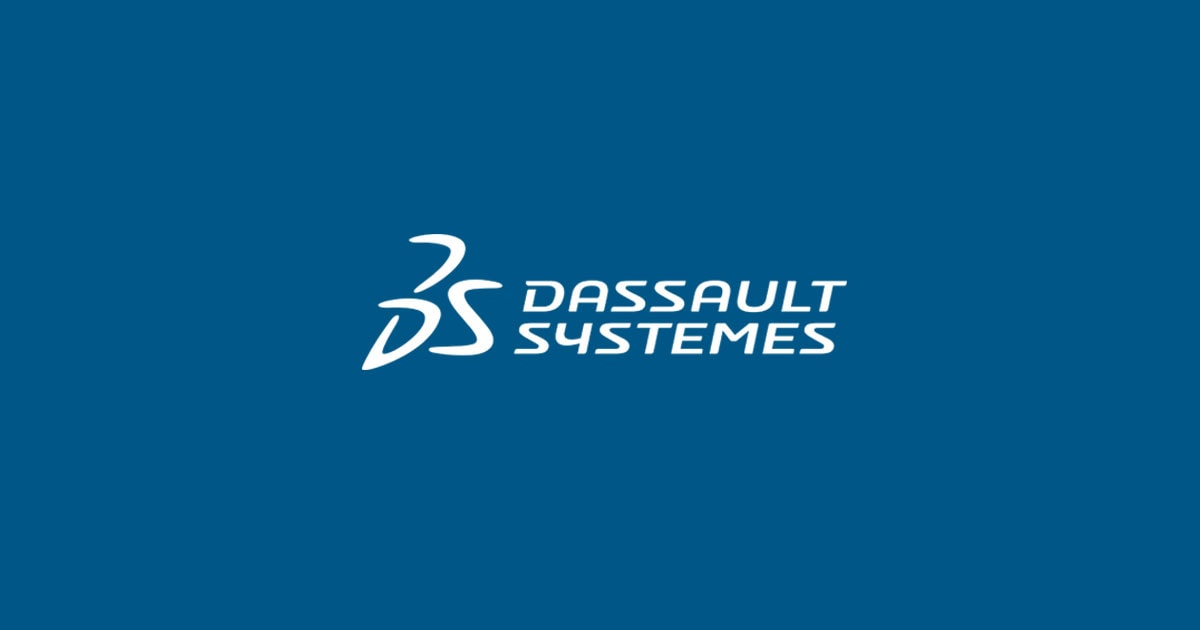June 28, 2018

# Part 1: Using Rimpull Tables in GEOVIA MineSched

The capability to use Rimpull tables to calculate truck velocities has been…Sifiso Buthelezi

The capability to use Rimpull tables to calculate truck velocities has been introduced in GEOVIA MineSched 9.2. This feature’s purpose is to improve the accuracy of calculating the truck’s cycle times by ensuring that they are aligned with Rimpull and Retard tables from the OEM’s (Original Equipment Manufacturer) handbook. This is an improvement as the truck’s velocities were inputs by the user in previous MineSched versions.

This blog aims to provide MineSched users with a background on Rimpull/Retard tables and explaining how they are used in MineSched 9.2.The CAT 973D is used as an example to explain the concept of Rimpull/Retard tables but it has to be emphasized that other trucks can be used in MineSched.

### When do you use Rimpull or Retard tables?

Rimpull tables are used when the truck is hauling upwards (increasing inclination/elevation) whether loaded or empty and the Retard tables are used to calculate the truck’s velocities when it is hauling downwards (decreasing inclination/elevation).

For example if a truck is hauling from the inside of an open pit to a stockpile or processing plant on the surface, like in Figure 1, the Rimpull tables will be used to calculate the truck’s velocity when it is loaded, as it will be hauling upwards, and the Retard tables will be used to calculate the truck’s velocities when empty, as it will be hauling downwards.

Now if mining occurs from a mountain or if the truck is hauling material from a higher to a lower elevation such as in Figure 2, then the truck will be hauling downwards loaded and upwards when empty. Hence the Retard tables will be used to calculate the loaded truck’s velocities and Rimpull tables will be used to calculate the empty truck’s velocity.

The information for both the Retard and Rimpull tables should be inserted in MineSched for loaded and empty trucks.

A few concepts that are used in both Rimpull and Retard tables are defined below:

• Rolling Resistance is the force that is resisting motion from occurring which is as a result of friction between the truck’s tire and the surface in which it is moving on.
• Grade the steepness of the slope that the truck will be required to travel and can be measured in degrees, as a percentage or as a ratio.
• Total Resistance is the sum grade and the rolling resistance. The total resistance is used for the Rimpull tables as when the truck is moving upwards it has to overcome more resistance.
•  Effective Grade is the grade minus the total resistance. The effective grade is used for the Retard tables, as the truck will have less resistance when it is hauling at a downward motion as it is hauling in the direction of motion.

### How to use the Rimpull table for Full/Loaded truck

Firstly, calculate the Total Resistance that the truck will need to overcome, which is the grade plus the rolling resistance;

For example, grade = 10% and rolling resistance = 2%, then

Total resistance = 10% + 2% = 12%, then draw a line from a total resistance of 12% to where it intercepts the dotted line for the loaded truck weight, illustrated in red in Figure 3.

1. From the loaded truck weight-total resistance interception, draw a horizontal line to intercept the highest gear illustrated in blue,
2. From the highest gear, draw a vertical line downwards and read the velocity that the truck will travel at that grade, illustrated in
3. Extend the blue line and read the Rimpull value in Kg x 1000, illustrated in yellow.

Note: The Rimpull value in Kg x 1000 will need to be multiplied by the gravitational acceleration (9.81 m/s-2) to obtain the frictional force that the truck will be required to overcome in order to travel at that particular grade and rolling resistance. This force will be used in future MineSched versions to calculate the fuel consumption.